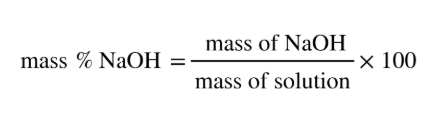# Problem: What mass of a 4.00% NaOH solution by mass contains 15.0 g of NaOH?

###### FREE Expert Solution
• This problem is looking for the total mass of the solution where we have a given % of NaOH and its mass on the solution
• Mass percent can be calculated:88% (409 ratings)###### Problem Details

What mass of a 4.00% NaOH solution by mass contains 15.0 g of NaOH?